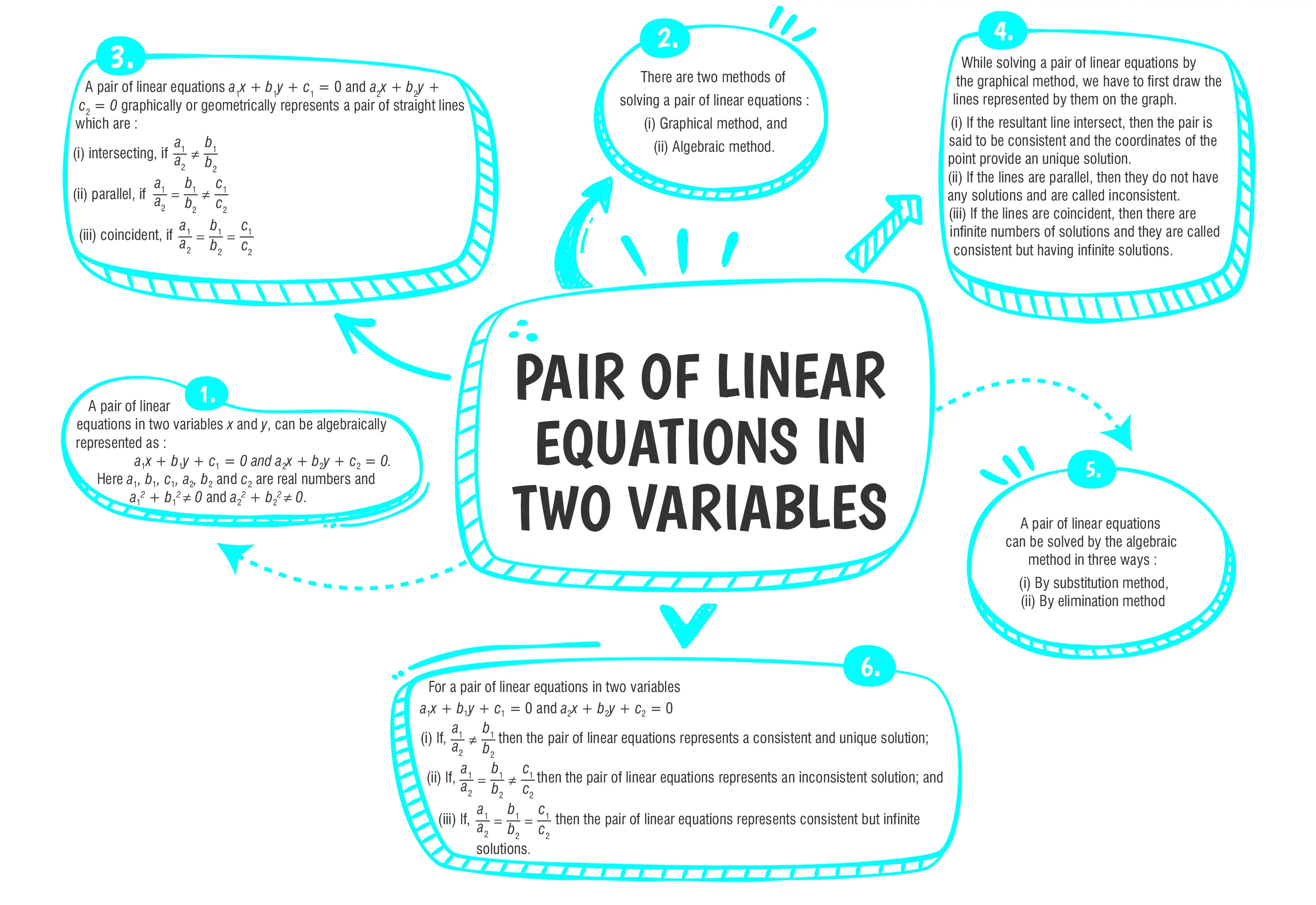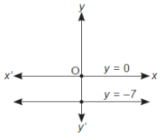Pair of Linear Equations in Two Variables

# Class 10 Maths Chapter 3 Pair of Linear Equation in Two Variables Important QuestionsHere are some important Class 10 Mathematics questions for Chapter 3, Pair of Linear Equations in Two Variables. These thoughtfully selected questions are designed to support students in their preparation for the CBSE Class 10 Mathematics Examination 2023-24. Practicing various question types will not only clarify doubts but also improve exam readiness. By solving these questions, students can boost their confidence in problem-solving and excel in the Pair of Linear Equations in Two Variables chapter.

## Introduction

In Chapter 3, "Pair of Linear Equations in Two Variables," we explore the graphical method of solving a system of two linear equations. The concept involves representing both equations graphically on the Cartesian plane and finding the point of intersection, which corresponds to the solution of the equations.

### What are pair of linear equations in two Variables?

Ans: A pair of linear equations in two variables is a set of two equations of the form:
ax + by = c
px + qy = r
where 'x' and 'y' are the variables, and 'a', 'b', 'p', 'q', 'c', and 'r' are constants with at least one of 'a' and ' B ' or 'p' and 'q' is not equal to zero.### Class 10 Pair of Linear Equations in Two Variables Important Questions and Answers

#### (a) Coincident(b) Parallel(c) Unique(d)  None of these

Ans. (a)

Explanation:
Given, 2x - 3y = 7 and (a + b) x - (a + b - 3)y = 4a+b
$${\Large\frac{a_1}{a_2}}={\Large\frac{b_1}{b_2}}={\Large\frac{c_1}{c_2}}\\[4.5bp]{\Large\frac{2}{a+b}}={\Large\frac{3}{a+b-3}}\\[4.5bp] ={\Large\frac{7}{4a+b}}\\[4.5bp] {\Large\frac{2}{a+b}} = {\Large\frac{7}{4a+b}}$$
8a + 2b = 7a +7b
a - 5b = 0
Hence lines are coincident.

#### (a) One solution(b) Two solution(c) Infinitely many solutions(d)  No solution

Ans. (d)

Explanation:
The given pair of equations y = 0 and y = - 7Graphically both lines are parallel and have no solution.

#### Q 3. Cost of 2 pens and 3 pencils together is ₹ 40 and the cost of 6 pens and 9 pencils together is ₹ 130. Express above statement in the form of linear equations.

Ans.     2x + 3y=40
and        6x + 9y=130

Explanation:
Given, cost of 2 pens and 3 pencils together is ₹ 40 and the cost of 6 pens and 9 pencils together is ₹ 130.
Let the cost of 1 pen be ₹ x and cost of 1  pencil be  ₹  y
Then,               2x + 3y=40
and                 6x + 9y=130

#### Q 4. Find whether the following system of equations is consistent or inconsistent :$${\Large\frac{3}{2}}x+{\Large\frac{5}{3}}y = 7 \text{ and 9x - 10y = 14.}$$

Ans. Consistent

Explanation:
We have,
$${\Large\frac{3}{2}}x+{\Large\frac{5}{3}}y - 7 = 0$$
and 9x - 10y - 14 = 0
$$\text{Here, }\space \space \space a_1 = {\Large\frac{3}{2}}, \space b_1 = {\Large\frac{5}{3}}, c_1 = -7$$
a2 = 9, b2 = - 10, c2 = - 14
$$\text{Thus, } {\Large\frac{a_1}{a_2}}={\Large \frac{3}{2×9}} = {\Large\frac{1}{6}}, {\Large\frac{b_1}{b_2}}={\Large\frac{5}{3(-10)}}=-{\Large\frac{1}{6}}\\[4.5bp] \text{Since, }\space\space \space {\Large\frac{a_1}{a_2}}\not= {\Large\frac{b_1}{b_2}}$$
So, a given system of equations is unique and it is consistent.

#### Q 5. Given the linear equation x – 2y – 6 = 0, write another linear equation in these two variables, such that the geometrical representation of the pair so formed is:(i) coincident lines(ii) intersecting lines

Explanation:
(i)
Given, x - 2y - 6 = 0
For lines to be coincident, the condition is:
$${\Large\frac{a_1}{a_2}}={\Large\frac{b_1}{b_2}}={\Large\frac{c_1}{c_2}}$$
Thus, one possible option can be:
2x - 4y - 12 = 0
Here, a1 = 1, b1 = - 2, c1 = - 6.
a2 = 2, b2 = - 4, c2 = - 12.
$${\Large\frac{a_1}{a_2}} = {\Large\frac{1}{2}};{\Large\frac{b_1}{b_2}}= {\Large\frac{-2}{-4}}={\Large\frac{1}{2}}; {\Large\frac{c_1}{c_2}}={\Large\frac{-6}{-12}}={\Large\frac{1}{2}}\\[4.5bp] ∴ {\Large\frac{a_1}{a_2}}={\Large\frac{b_1}{b_2}}={\Large\frac{c_1}{c_2}}$$
So, it shows coincident lines.
(ii) Given, 2x - 2y - 6 =0
For intersecting  lines
$${\Large\frac{a_1}{a_2}}\not={\Large\frac{b_1}{b_2}}$$
Thus, one possible can be:
2x - 7y - 13 = 0
Here, a1 = 1, b1 = - 2, c1 = - 6.
a2 = 2, b2 = - 7, c2 = - 13.
$${\Large\frac{a_1}{a_2}}= {\Large\frac{1}{2}}, {\Large\frac{b_1}{b_2}} = {\Large\frac{-2}{-7}}={\Large\frac{2}{7}}\\[4.5bp] ∴ {\Large\frac{a_1}{a_2}} ≠ {\Large\frac{b_1}{b_2}}$$
So, they represent intersecting lines.### CBSE Class 10 Maths Chapter wise Important Questions

Chapter No. Chapter Name
Chapter 1 Real Number
Chapter 2 Polynomials
Chapter 3 Pair of Linear Equations in Two Variables
Chapter 5 Arithmetic Progressions
Chapter 6 Triangles
Chapter 7 Coordinate Geometry
Chapter 8 Introduction to Trigonometry
Chapter 9 Some Applications of Trigonometry
Chapter 10 Circles
Chapter 11 Areas Related to Circle
Chapter 12 Surface Areas and Volumes
Chapter 13 Statistics
Chapter 14 Probability

#### Conclusion

If you are seeking additional practice and a deeper grasp of the concepts covered in the chapter, oswal.io offers a wide-ranging collection of questions that will help you gain a more comprehensive understanding of the topic. By exploring their extensive question bank, you can reinforce your knowledge, improve your problem-solving abilities.

#### Q1: What are the algebraic methods to solve a pair of linear equations in two variables?

Ans: The two common algebraic methods are the substitution method and the elimination method.

#### Q2: How are pairs of linear equations in two variables solved graphically?

Ans: Graphical method involves plotting both equations on a Cartesian plane as lines and finding the point of intersection, which represents the solution to the system.

#### Q3: Can a pair of linear equations have more than one solution?

Ans: Yes, if the two lines representing the equations coincide (overlap), the system has infinitely many solutions.

#### Q4: What is the graphical method of solving a pair of linear equations?

Ans: The graphical method involves representing each equation on the Cartesian plane as a straight line by plotting its intercepts or using the slope-intercept form. The solution to the pair of equations is the point of intersection of these lines on the graph.

#### Q5: Is it possible for a pair of linear equations to have no solution?

Ans: Yes, if the two lines representing the equations are parallel and do not intersect, the system has no solution.

###### Copyright 2022 OSWAL PUBLISHERS Simplifying ExamsPhone:  (+91) 78959 87722
Mail: support@oswalpublishers.in